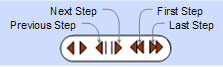Morley's Triangle and Center. Interactive Geometry Software.. Level: High School, SAT Prep, CollegeThe three points of intersection of the adjacent angle trisectors of any triangle ABC form an equilateral triangle DEF called Morley's triangle. Morley center is the intersection point of AE, BF and CD.

Hint to interact with the figure (below): Click the red button () on the figure to start the animation. Drag points A, C and line AC to change the figure. Press P and click the left mouse button to start the step by step construction.

Intersection of two lines is the point at which they concur.

Angle trisector
are two line that divide an angle in three equal parts.

Equilateral triangle is a triangle with all three sides of equal length.

Frank Morley (1860 – 1937) was a leading mathematician, known mostly for his research into algebra and geometry. Among his accomplishments was his discovery and proof of the celebrated Morley's trisector theorem in elementary plane geometry, one of the most striking theorems in triangle geometry. Frank Morley was a member of Haverford College's Department of Mathematics in the early part of the twentieth century.

Dynamic Geometry: You can alter the figure above dynamically in order to test and prove (or disproved) conjectures and gain mathematical insight that is less readily available with static drawings by hand.

This page uses the TracenPoche dynamic geometry software and requires Adobe Flash player 7 or higher. TracenPoche is a project of Sesamath, an association of French teachers of mathematics.

Instruction to explore the illustration above:

• Animation. Click the red buttonto start/stop animation

• Manipulate. Drag points A and C, and line AC to change the figure.

• Step-by-Step construction. Press P and click the left mouse button on any free area to show the step-by-step bar and click 'Next Step' button () to start the construction step-by-step:Hide the step-by-step bar by using again the combination P + click left mouse.Home | Geometry | Dynamic Geometry | TracenPoche Index | Email | By Antonio Gutierrez Last updated: August 1, 2009